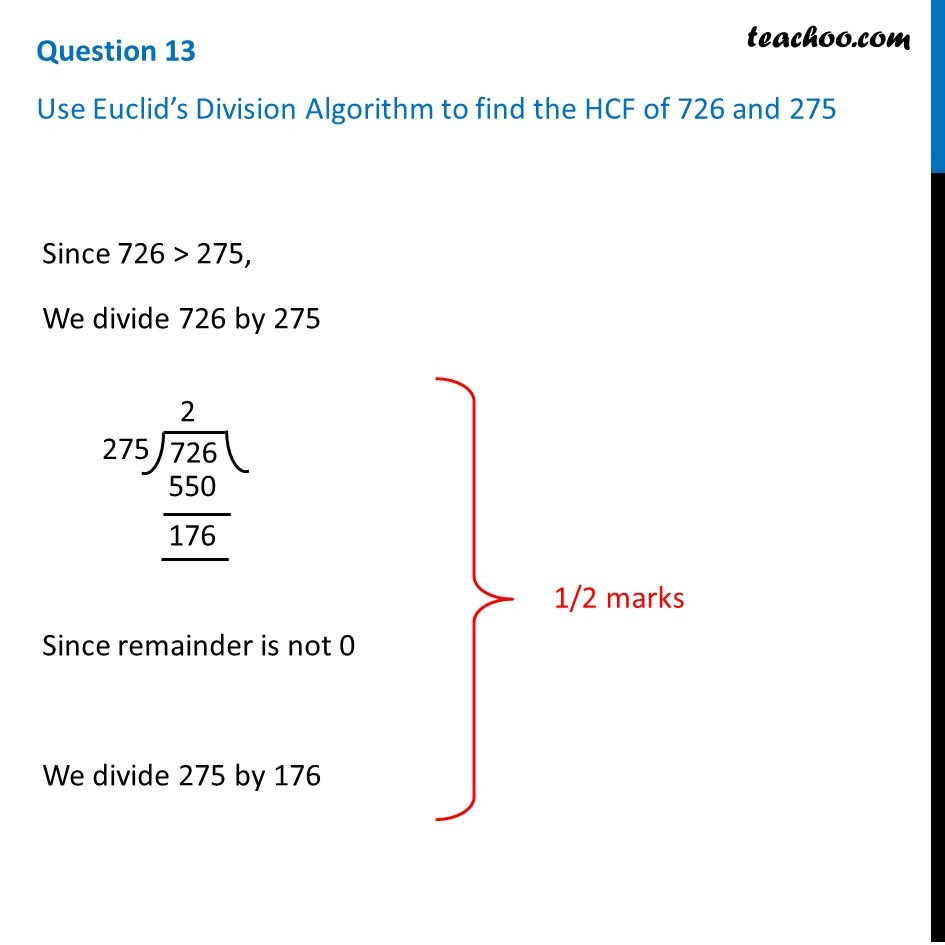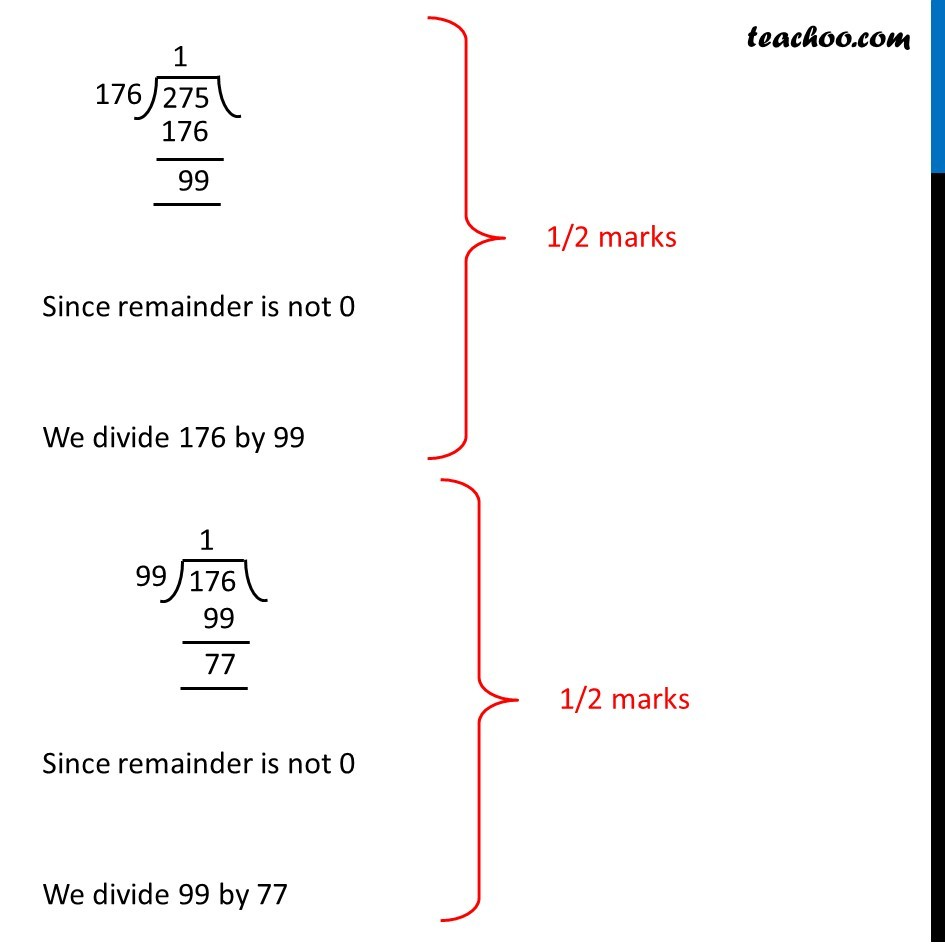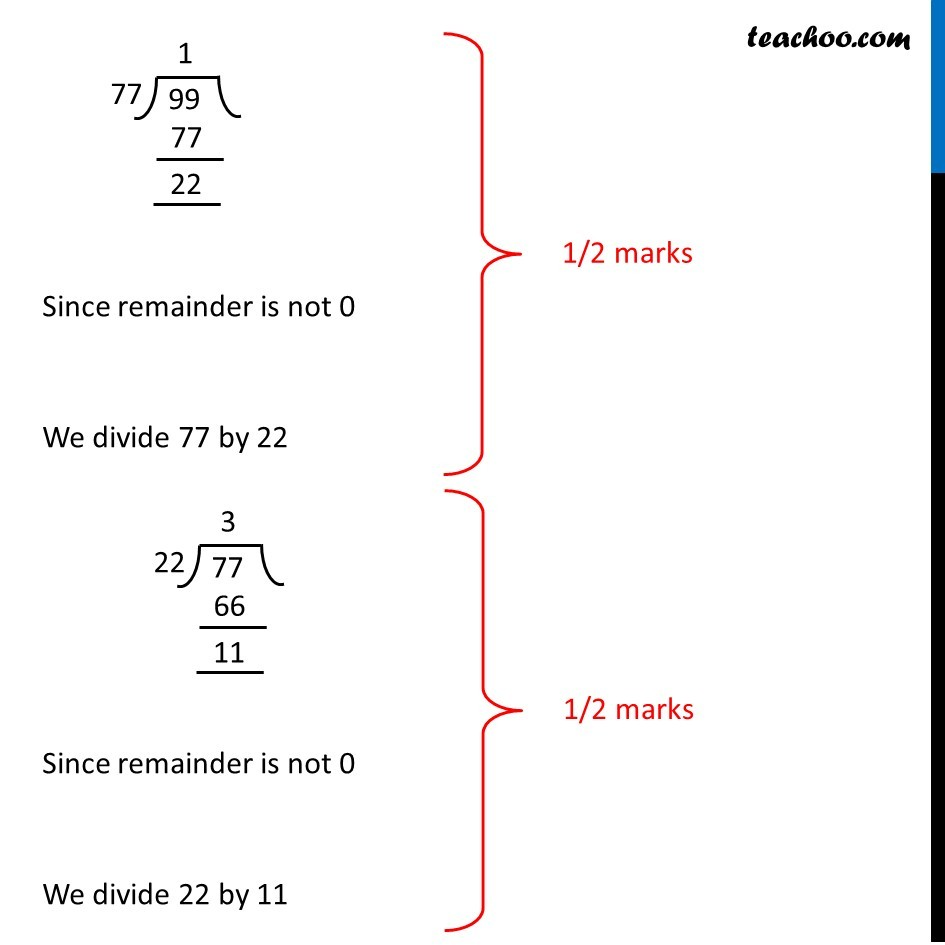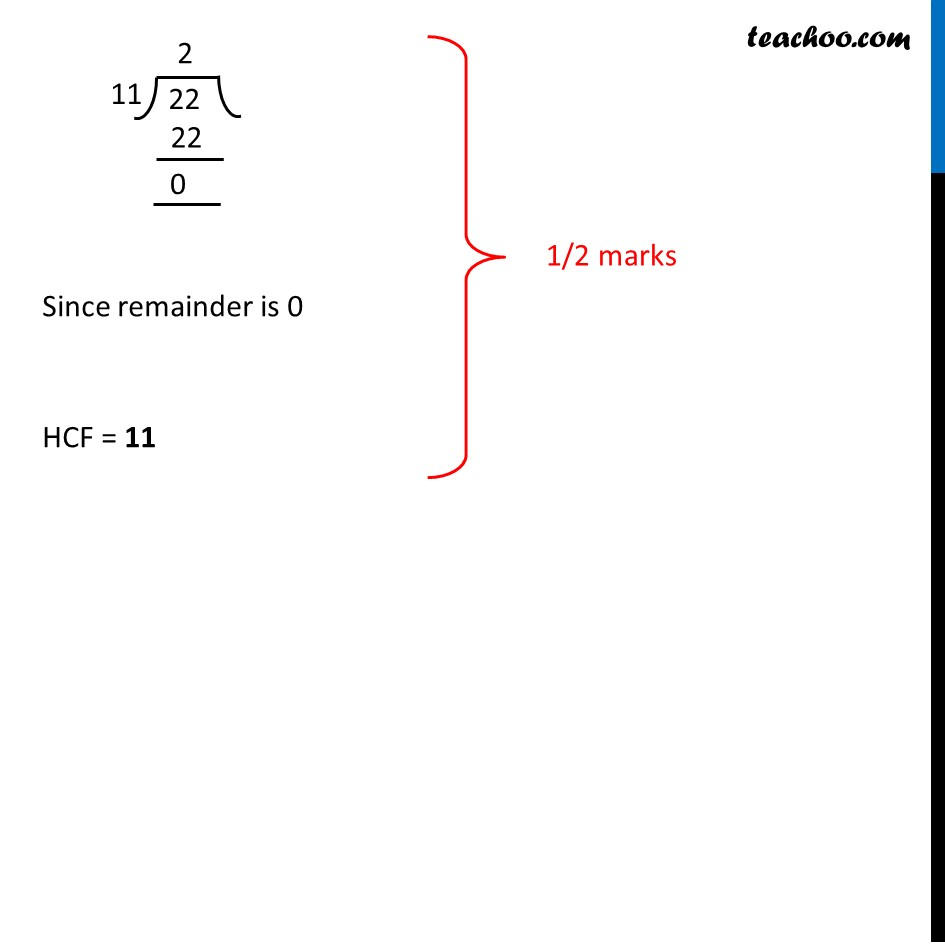CBSE Class 10 Sample Paper for 2019 Boards

Class 10
Solutions of Sample Papers for Class 10 Boards

Question 13

Use Euclid’s Division Algorithm to find the HCF of 726 and 275Learn in your speed, with individual attention - Teachoo Maths 1-on-1 Class

### Transcript

Question 13 Use Euclid’s Division Algorithm to find the HCF of 726 and 275 Since 726 > 275, We divide 726 by 275 Since remainder is not 0 We divide 275 by 176 1/2 marks Since remainder is not 0 We divide 176 by 99 1/2 marks Since remainder is not 0 We divide 77 by 22 1/2 marks Since remainder is not 0 We divide 22 by 11 Since remainder is not 0 1/2 marks We divide 99 by 77 1/2 marks Since remainder is 0 HCF = 11 1/2 marks